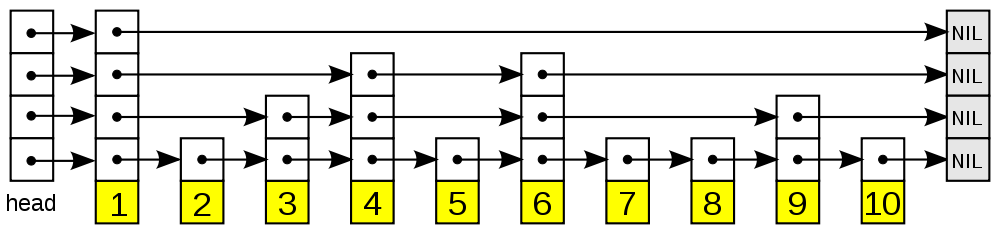# 跳转表 (skiplist) 的期望分析

3 分钟阅读

2019 年 10 月 27 日

## Intro## 空间性能

(这个结果一点都不意外: 从 1 开始以 $p$ 的增长率增长到 $N$ 的结果就是这个. 不过一个比较严格的计算过程略微有点费劲, 容易出现把求和拆成两个发散和的错误)

## 时间性能

1. 总的时间复杂度 $\mathcal O(\log N)$
2. 最佳的概率 $p^*$ 取决于 $\xi$ : $p^*(\ln 1/p^* - 1) = \xi - 1$
• 特别的当 $\xi = 1$ 时, $p^* = 1/e$ 是最优解;
• 当 $\xi\to 0$ 时搜索耗时关于 $p$ 单调递减.

## Python 实现

from random import randrange as rand, random as drand
def __init__(self, val=None, right=None, below=None):
self.val = val
self.right = right
self.below = below

class SkipList:
def __init__(self):

def _search(self, val):
prevs = []
while c.below:
while c.right and c.right.val < val:
c = c.right
prevs.append(c)
c = c.below
while c.right and c.right.val < val:
c = c.right
return c, prevs

def _insert(self, val, c, prevs):
c = c.right
# while rand(2):
while drand() > 0.632:
# 0.632 = 1-1/e
if prevs:
last = prevs.pop()
else:

def _delete(self, val, c, prevs):
assert c.right.val == val
while c.right and c.right.val == val:
c.right = c.right.right
if prevs:
c = prevs.pop()
else:
break
# clean empty layers

def search(self, val):
c, _ = self._search(val)
return c.val, c.right.val if c.right else None

def insert(self, val):
self._insert(val, *self._search(val))

def delete(self, val):
self._delete(val, *self._search(val))